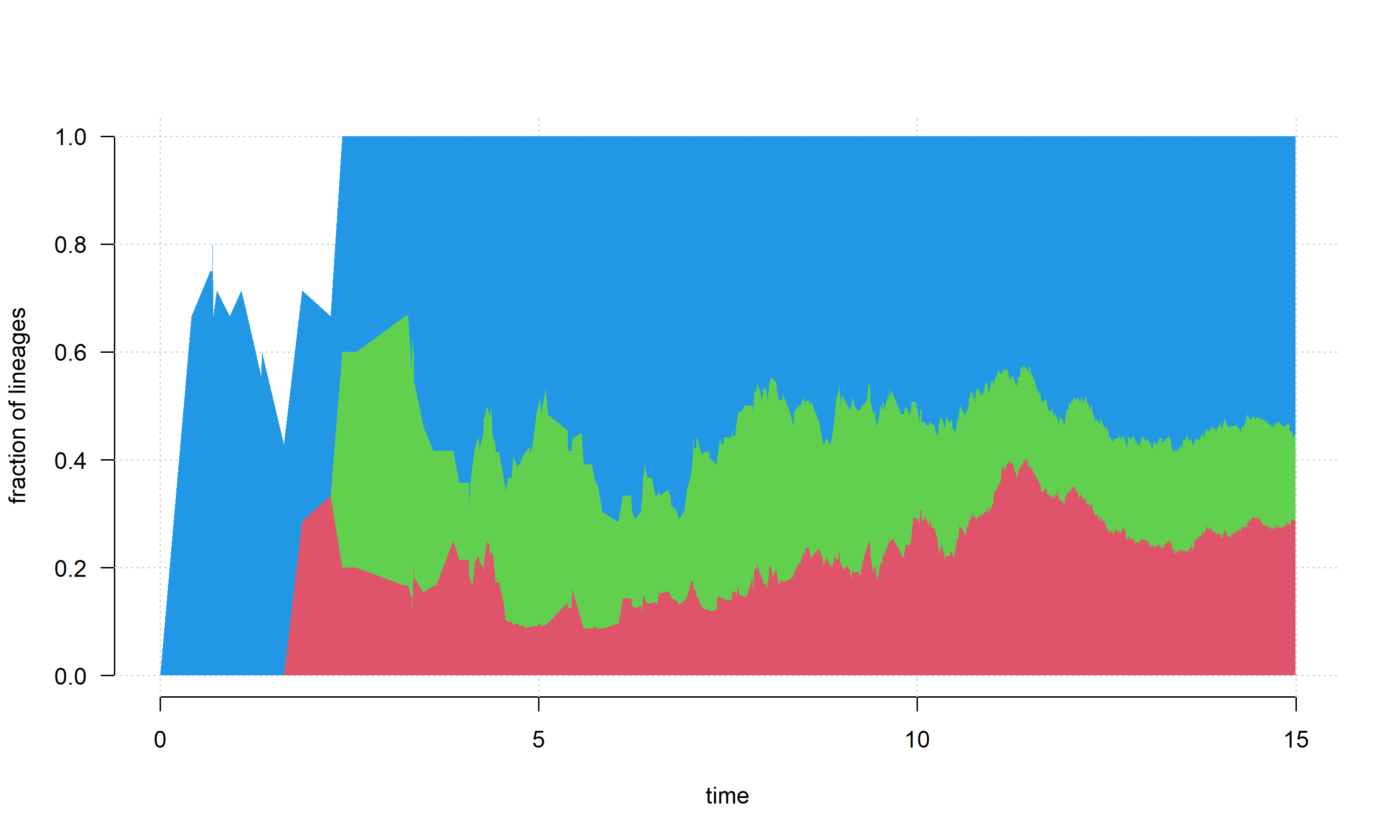## Saturday, October 28, 2023

### Visualizing lineage accumulation on the tree by clade (or mapped regime)

The other day, an R-sig-phylo list member asked the following question:

“I was wondering if there was any way to color-code a lineage-through-time plot, to highlight the proportion of taxa at specific intervals that belong to a particular clade. I.e., an LTT plot of tetrapod diversity through time, and I want to highlight the number of lineages at any one point in time that are chondrichthyans, sarcopterygians, lissamphibians, etc. (see attached fig. as an example). I figured I can do this by asking R to return all lineages that are descendants of a specific node, but am not sure what functions I can use to convert the dated tree into an object that can be read into ggplot.”

Will Gearty had a good suggestion, which was to simply map the clades of interest on the tree as different regimes in a `"simmap"` object, and then use existing phytools functionality to create an `"ltt"` object separated by regime.

This is a great idea… the only catch is that the current CRAN version of `ltt.simmap` (it turns out, by virtue of using `ape::branching.times` internally) does not support non-ultrametric trees. This is now fixed on GitHub.

With the newest version of phytools installed, here’s a simplified demo of how one might go about implementing Will’s suggestion.

``````library(phytools)
packageVersion("phytools")
``````
``````##  '1.9.24'
``````

``````tree
``````
``````##
## Phylogenetic tree with 1162 tips and 1161 internal nodes.
##
## Tip labels:
##   t2, t27, t28, t29, t24, t19, ...
##
## Rooted; includes branch lengths.
``````
``````par(mar=rep(0.1,4))
plot(tree,type="tidy",show.tip.label=FALSE)
``````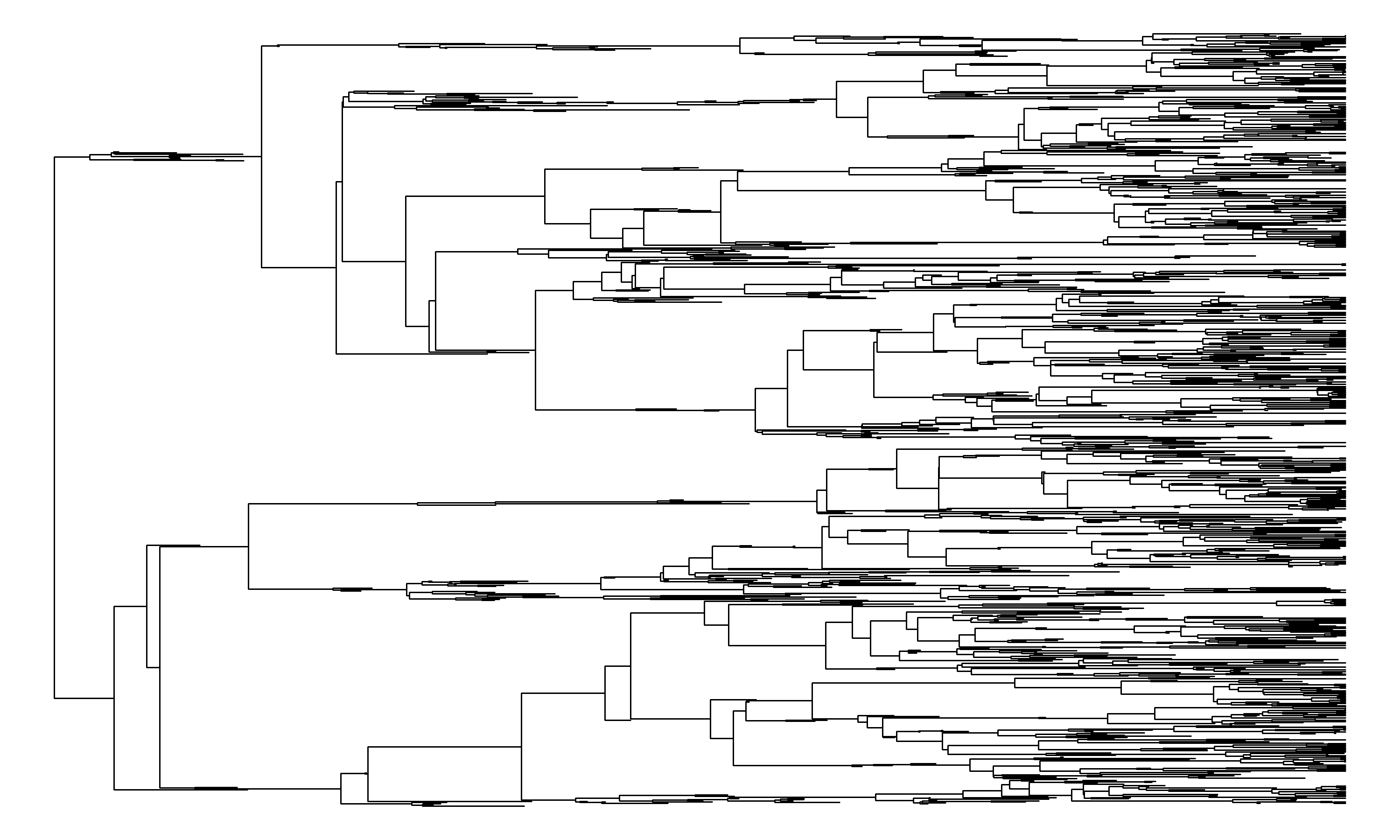This tree has the following total lineage accumulation curve.

``````plot(ltt(tree,plot=FALSE),las=1,bty="n",log.lineages=FALSE,
log="y")
grid()
``````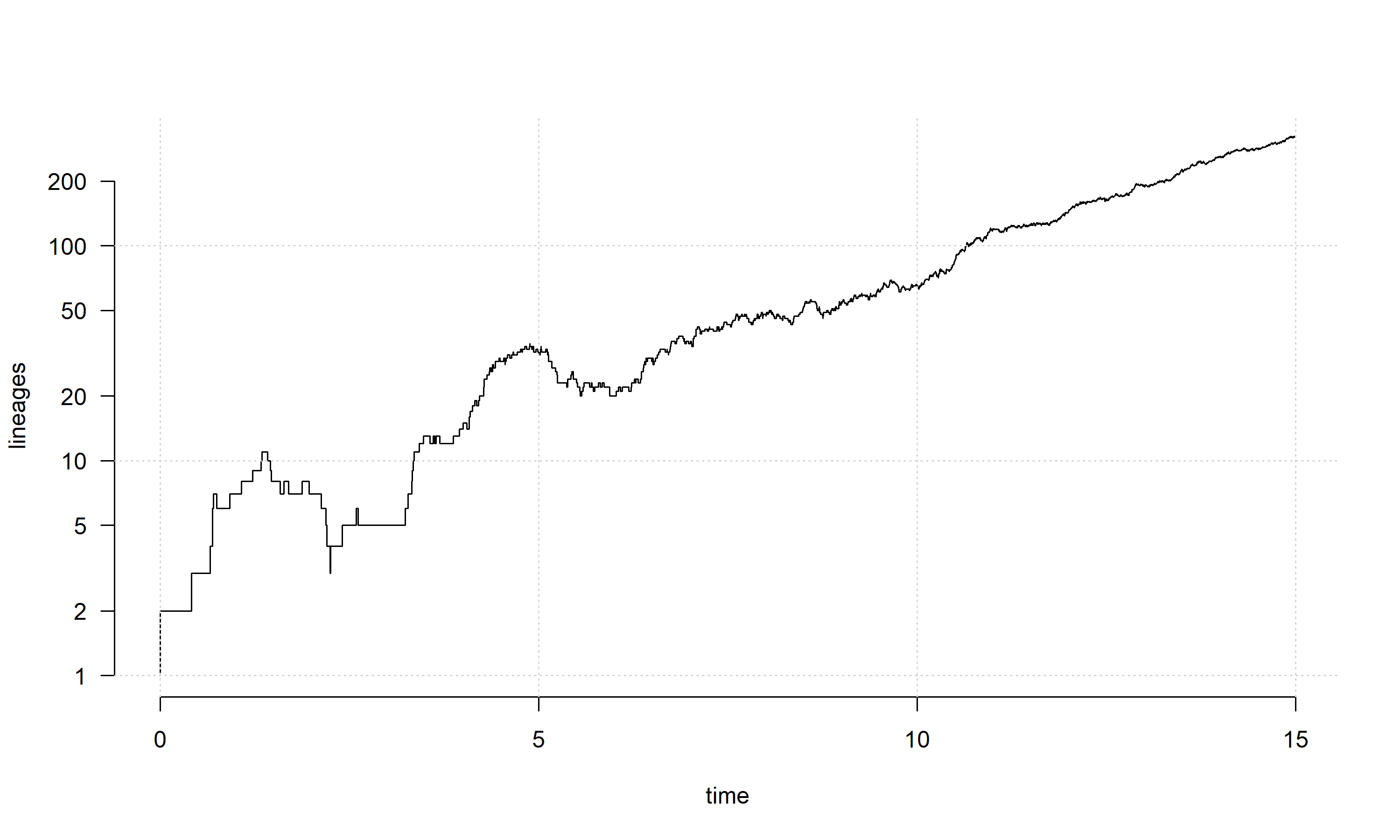Now, according to the R phylogenetics user question, we want to split this lineages accumulation out by clade.

For the purposes of our example, let’s focus on three clades that feature both extinct & extant descendants, and all originate relatively early in the tree.

These nodes have the numerical indices 1167, 1468, and 1706 – which (given a set of tip labels) I could’ve found using (for example) `getMRCA`.

``````nodes<-c(1167,1468,1706)
par(mar=rep(0.1,4))
cols<-palette()[2:4]
plot(tree,type="tidy",show.tip.label=FALSE)
nodelabels(node=nodes,pie=diag(rep(1,3)),
piecol=cols,cex=0.5)
``````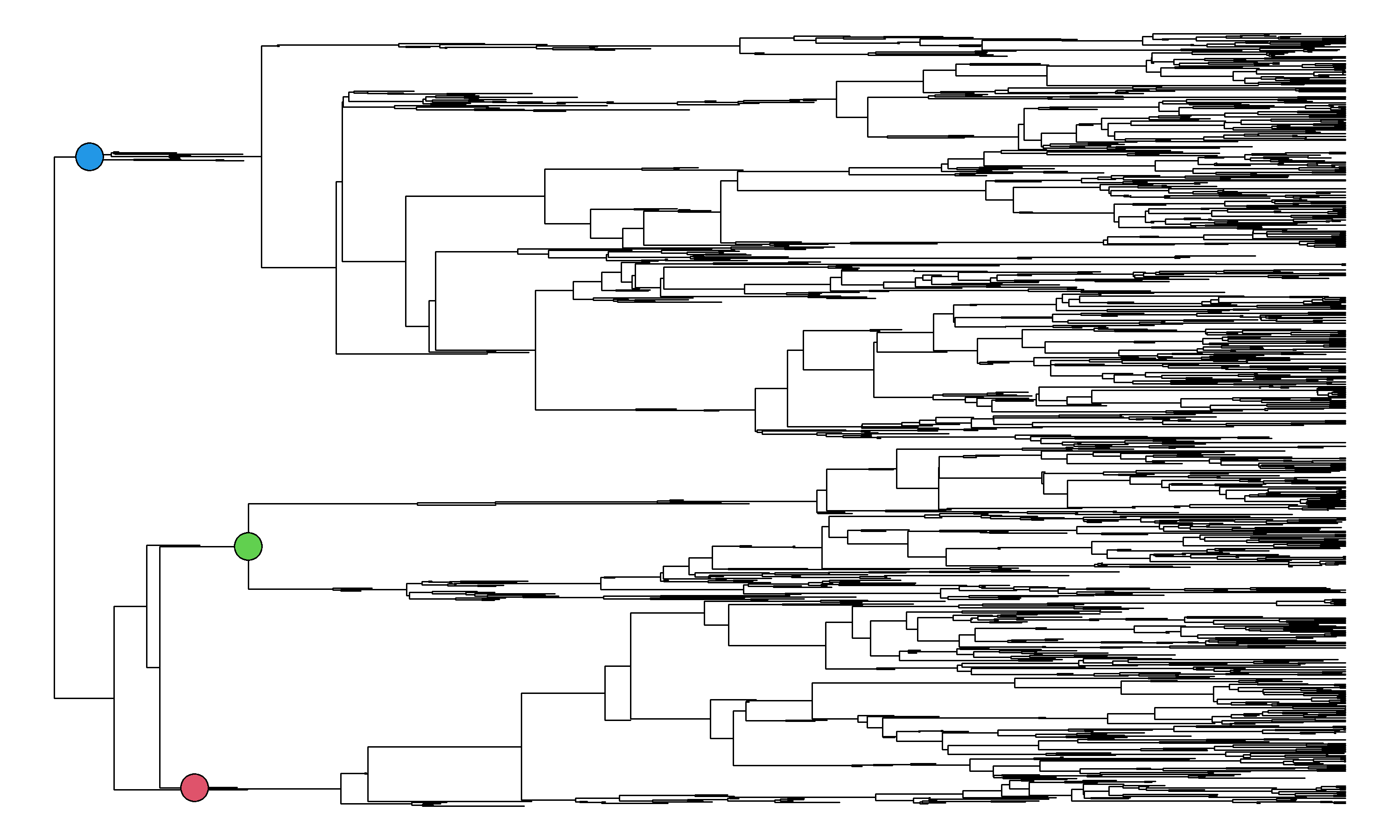Our next step is to map each of these three clades as regimes in a `"simmap"` object, which we can do easily based on their node numbers using `phytools::paintSubTree` as follows.

``````painted_tree<-tree
for(i in 1:length(nodes)) painted_tree<-paintSubTree(painted_tree,
nodes[i],i,anc.state="0")
``````

Just to make sure that we got our regimes correct, let’s plot our tree. (Since I like the “tidy” layout that I showed before, but this is not implemented in phytools, we can use a trick I posted previously on my blog to get it.)

``````cols<-setNames(palette()[1:4],0:3)
plot(tree,type="tidy",show.tip.label=FALSE,plot=FALSE)
tips<-get("last_plot.phylo",envir=.PlotPhyloEnv)\$yy[1:Ntip(tree)]
``````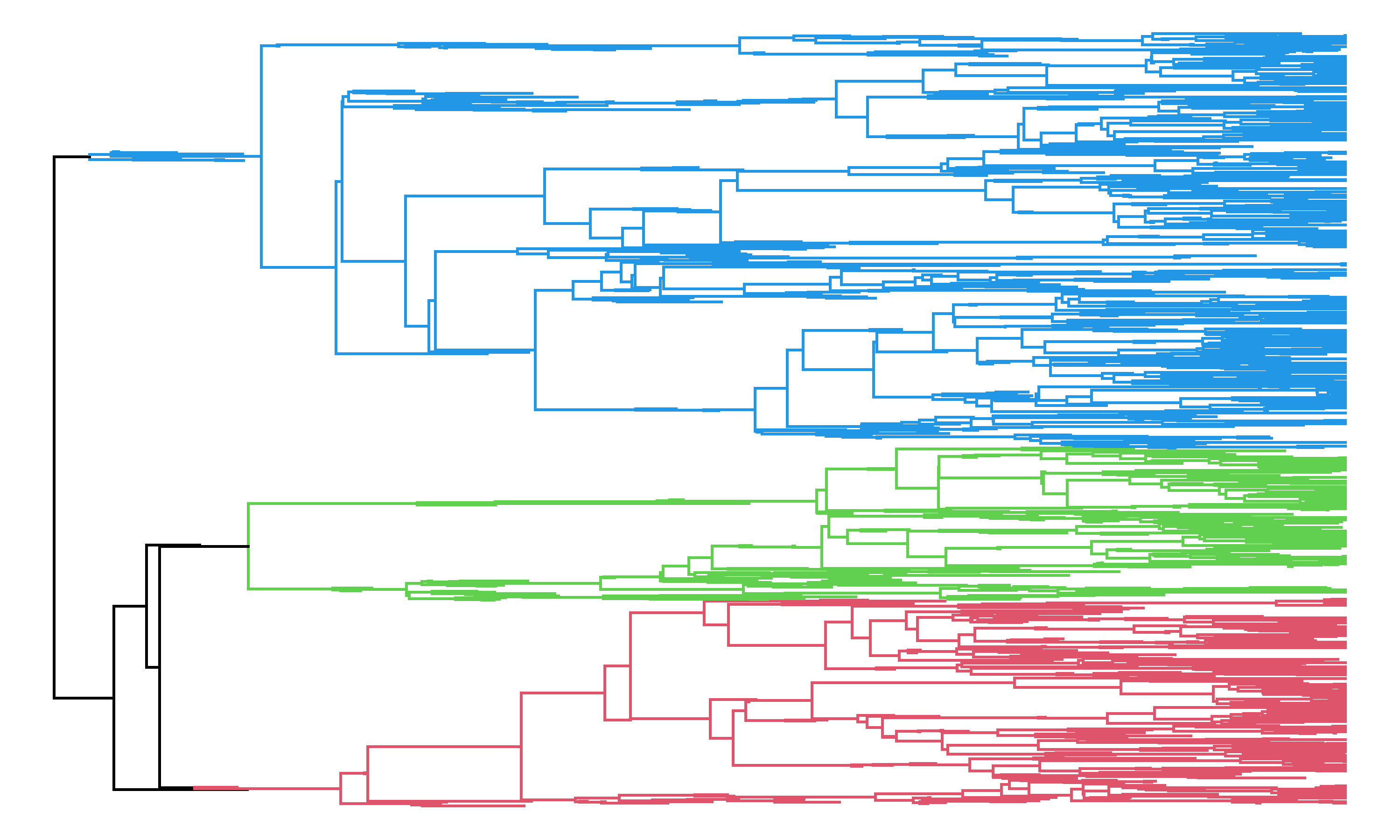Now, let’s just run `ltt` on this new object, as follows.

``````obj<-ltt(painted_tree,show.total=FALSE,colors=cols,ylim=c(0,200),
bty="n",las=1)
grid()
``````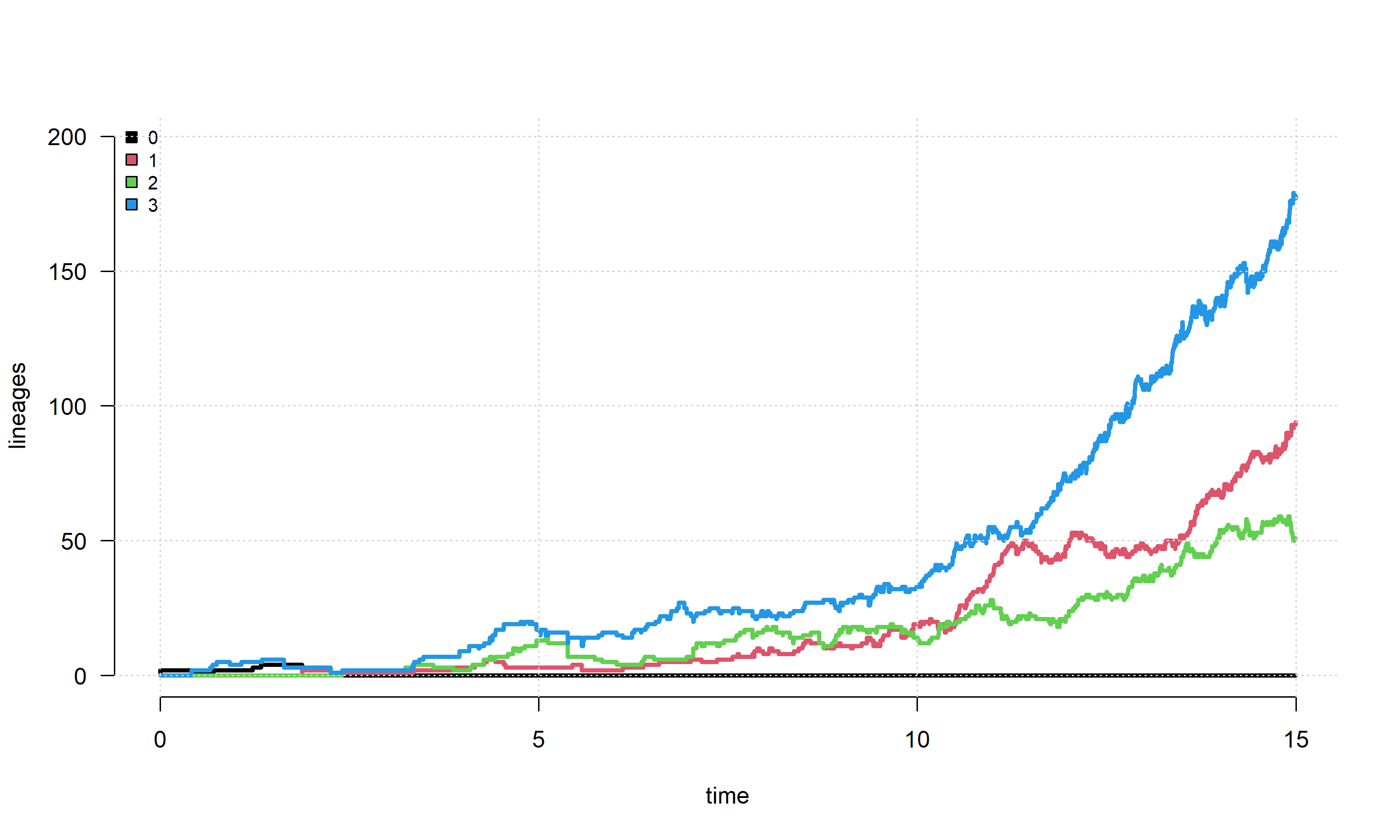We may want to show the accumulation of lineages in each clade or regime at each time point on the tree from the root to the present day.

``````cumul_ltt<-t(apply(obj\$ltt,1,function(x) cumsum(c(0,x[1:4]))))
plot(NA,xlim=range(obj\$times),ylim=range(cumul_ltt),
xlab="time",ylab="cumulative lineages",bty="n",las=1)
grid()
for(i in 2:ncol(cumul_ltt))
polygon(x=c(obj\$times,obj\$times[length(obj\$times):1]),
y=c(cumul_ltt[,i-1],cumul_ltt[nrow(cumul_ltt):1,i]),col=cols[i-1],
border="transparent")
``````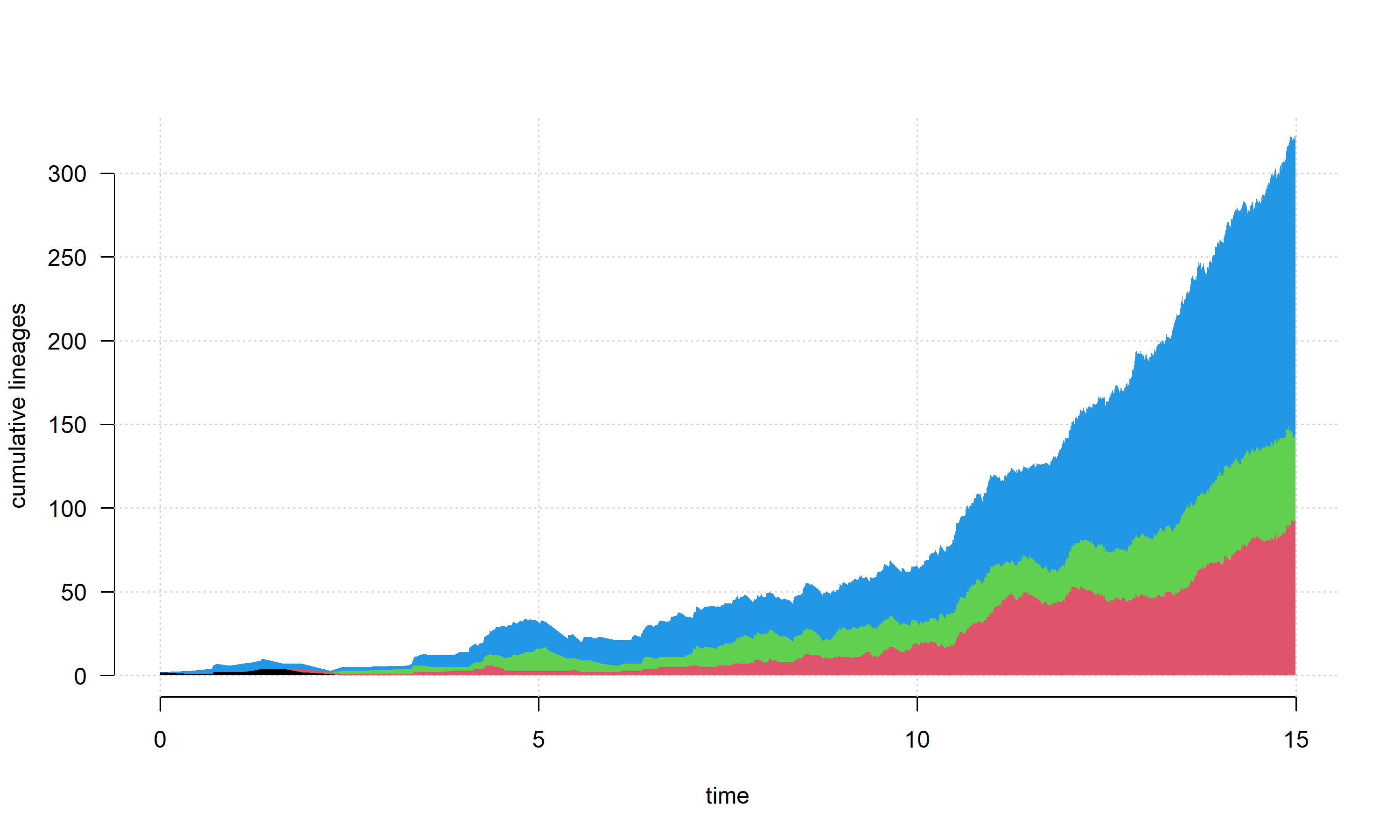Lastly, we could desire to show the proportion of lineages in each of the categories. Here, I’ll exclude the root “regime” since we’re interested in the diversity of each of our three identified clades.

``````prop_ltt<-t(apply(obj\$ltt,1,function(x) cumsum(c(0,x[2:4]))/x))
plot(NA,xlim=range(obj\$times),ylim=range(prop_ltt),
xlab="time",ylab="fraction of lineages",bty="n",las=1)
grid()
for(i in 2:ncol(prop_ltt))
polygon(x=c(obj\$times,obj\$times[length(obj\$times):1]),
y=c(prop_ltt[,i-1],prop_ltt[nrow(prop_ltt):1,i]),col=cols[i],
border="transparent")
``````